# FAQs: Desmos Graphing Calculator

### Below are frequently asked questions from Desmos users just like you!

How do I plot a point?

You can plot points one at a time, a few on a line (separated by commas), or all in a table...whichever you prefer. To get started try typing in a coordinate pair in the expression list such as (2,7).

How do I graph a line or a curve?

Enter the equation of the line you would like to see graphed. Try $$y = 2x + 3$$. You can also enter equations that are not solved for $$y$$. Try something like $$3x - 4y = 2$$.

How do I graph a dotted line instead of a solid one?

To change the color or style (dotted, dashed, line thickness, etc.) of a curve, long-hold the colored icon to the left of the expression. Clicking the icon will hide or show the expression.

How do I change the color or style of the graph?

To change the color or style of an expression, long-hold the colored icon to the left of the expression. Clicking the icon will hide or show the expression.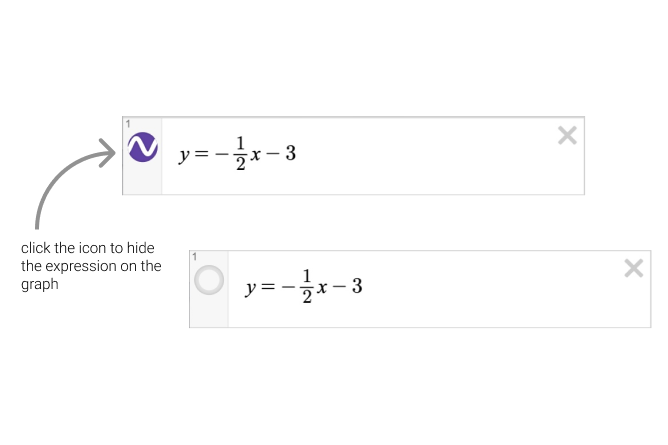Clicking and holding the icon next to the expression will open the color and style menu.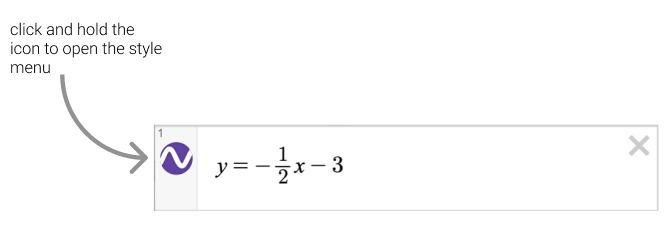Lines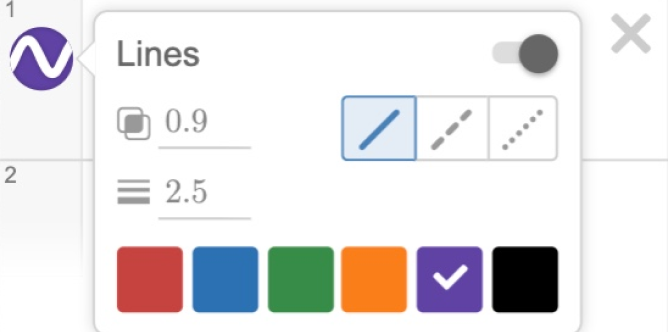Tables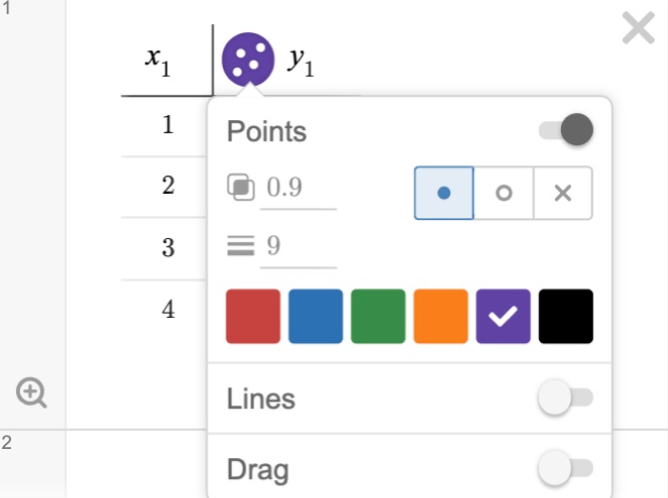Points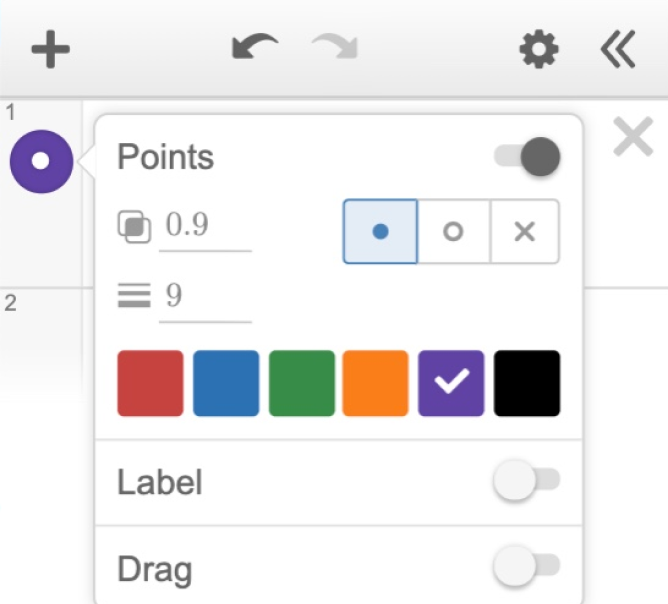You have the option to change the opacity and thickness of lines, points and labels by adjusting these values in the color/style settings.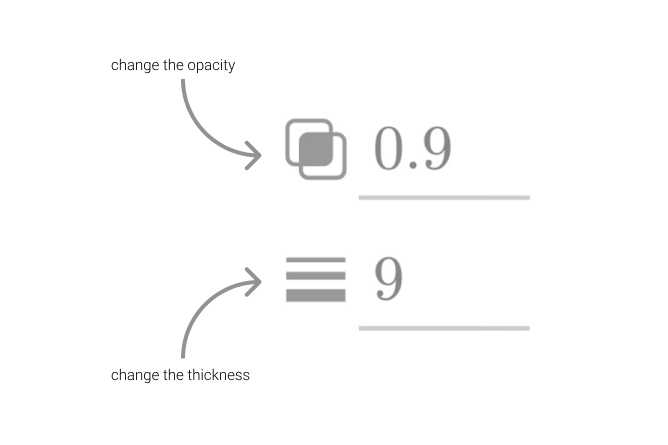How do I show only part of a curve on the graph?

To limit the domain or range ($$x$$ or $$y$$ values of a graph), you can add the restriction to the end of your equation in curly brackets {}. For example, $$y = 2x \{1 \lt x \lt 3\}$$ would graph the line $$y = 2x$$ for $$x$$ values between 1 and 3.

You can also use range restrictions. For example, $$y=x^{2} \{y\gt3\}$$ would only show the part of the parabola where $$y$$-values are greater than 3. Desmos also allows for multiple restrictions to the same expression line regardless of what parameter is being restricted. For example, $$x^{2}+y^{2}=20 \{x\lt0\}\{y\gt0\}$$ would only show the circle graphed in the second quadrant.

How do I enter a fraction in the expression line?

To enter a fraction, try using the "/" symbol on the device keyboard. Or, you can use the division symbol from the Desmos Keypad (lower left) to create a fraction.

How do I Undo and Redo?

If you accidentally delete a large expression or table, your normal keyboard commands for undo and redo (CTRL/COMMAND-Z and CTRL/COMMAND-SHIFT-Z) will work.

There are also quick arros for Undo and Redo at the top of the expression list.

How do I display my graph better?

To adjust the viewing window, you can use the plus and minus signs located in the top right of your window to zoom in and out.

You can also manually adjust the domain and range of the viewport. To do so, click on the wrench to open the Graph Settings menu and choose which axis you'd like to adjust.

If you have a table in the expression window, you can click on the Zoom Fit icon (looks like a magnifying glass at the bottom of the table) to fit the window to your data.

How do I change my $$x$$- and $$y$$-axes?

Click the graph settings wrench in the upper right. Here you have options to change which values are shown on each axis as well as the step of the axis.

How do I label my $$x$$- and $$y$$-axes?

Click the graph settings wrench in the upper right. Here you have the option to label your axes.

How do I show only the $$x$$- and $$y$$-axes and not the grid?

Click the graph settings wrench in the upper right. Here you have options to include a grid or minor gridlines.

How do I change from radians to degrees?

Click the graph settings wrench in the upper right, you'll see a radians/degrees toggle.

How do I find the intersection point of two or more curves?

If you click a curve or expression, you'll see gray dots appear at interesting points including maximums, minimums, intercepts, and intersections. Click on a gray dot to open the coordinates at that point - click the point again to hide the coordinates. You can also trace along a function by clicking and dragging along the curve.

Note: Your equation needs to be solved for y in most cases for this to work.

How do I find the minimum or maximum of a curve?

If you click a curve or expression, you'll see gray dots appear at interesting points including maximums and minimums. Click on a gray dot to open the coordinates at that point - click the point again to hide the coordinates. You can also trace along a function by clicking and dragging along the curve.

How do I find $$x$$- and $$y$$-intercepts?

If you click a curve or expression, you'll see gray dots appear at interesting points including the $$x$$- and $$y$$-intercepts. Click on a gray dot to open the coordinates at that point - click the point again to hide the coordinates. You can also trace along a function by clicking and dragging along the curve.

How do I add an image to my graph?

To add an image to your graph, you can use the Add Item menu (plus sign in the top left of the expression list) and scroll down to Image. You can also drag an image from your desktop directly into the expression list.

How do I shade in part of my graph?

Use inequalities to automatically shade above or below lines and curves.

For more intricate graphs, you can also use inequalities with restrictions to shade only selected parts of the graph. For example $$y\gt$$$$x$$$$\{y\lt4\}$$ would shade in above the line $$y=x$$, but only for $$y$$-values that are less than 4.

How do I make a Table in the Graphing Calculator?

To make a table in the Desmos graphing calculator simply type table, or use the Add Item menu (plus sign in the top left of the expression list) and scroll down to Table.

Tables can also be created from a single point: (1,0)

A list of points separated by commas: (1, 0), (2, 1),(3, 2), (4, 3)

Or, from function notation: f(x) = sin(x)

For each instance above, click the gear icon at the top of the expression list (Edit List) and then click the table icon next to the expression (Convert to Table) or (Create Table). Learn More.

How do I make Lists in the Graphing Calculator?

You can make lists in Desmos using square brackets like this: l = [1, 2, 3, 4, 5]

You can create lists with evenly spaced elements. [1,...,10] is a list of the integers between 1 and 10. [1,3,...,11] is a list of the odd integers between 1 and 11. You can use lists anywhere in expressions that you would use a number.

How do I graph piecewise functions?

To write a piecewise function, use the following syntax: y = {condition: value, condition: value, etc.} For example:See an example of the graph here .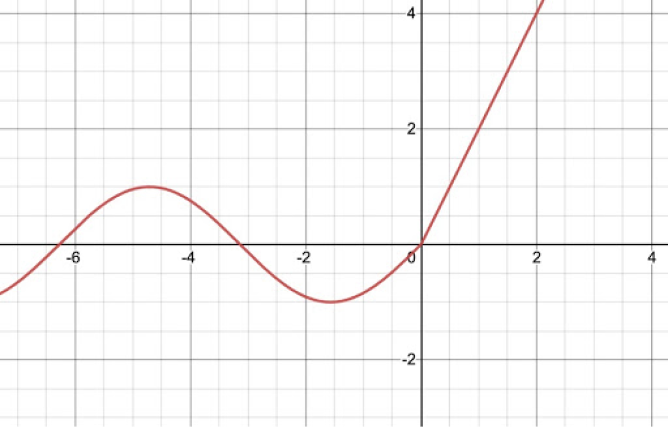How do I connect points?

Create a table using your point values. Next, click and long-hold the icon in the y-column header. To connect points, toggle the line feature on. Note that using the line feature from a table will connect the points discretely. To plot a continuous curve, plot the function in a separate line and then use the 'create table' option described under 'How do I make a table in the graphing calculator?'

How do I solve an equation using the graphing calculator?

To solve your equation graphically, graph both sides in separate expression lines. By clicking on the intersection point(s) between the two lines, you'll find the solution as the x-value(s) of the intersection point(s). See an example here.

How do I print my graph?

To print your graph, you'll need to access Desmos in the browser. Unfortunately, printing isn't available on mobile devices. Start by clicking the Share button and choosing Print.

A dialog box will pop up with a preview of the printout. An image of your graph will print on the first page and the expression list will print on the next pages. If you'd like for the graph image to fill the page, try printing in landscape mode rather than portrait.

How do I graph in 3D?

While Desmos doesn’t have a dedicated 3-D Graphing Calculator yet, you can mimic 3-D graphing using f(x,y) notation. Click here to see an example of it in action.

You can also watch one of our #DesmosLive webinars where our Graph Specialist Suzanne shared some of her favorite 3D Graphing tricks.

Why does my graph look strange (unresolved detail)?

Did you enter an expression in the Graphing Calculator and the resulting graph lacked some detail that you expected to find?

Sometimes the calculator detects that an equation is too complicated to plot perfectly in a reasonable amount of time.

When this happens, the equation is plotted at lower resolution.

How do I share my graph with someone else?

If you're logged into your account at desmos.com/calculator, you can press the "Share" button in the upper right (looks like an arrow), and you’ll see some options for sharing your graph.

How do I use the Reverse Contrast setting?

Click the graph settings wrench in the upper right, you'll see a display settings at the top. You want to turn on ‘Reverse Contrast’.